Work & Heat Transfer

# Work & Heat Transfer - Notes | Study Mechanical Engineering SSC JE (Technical) - Mechanical Engineering

 1 Crore+ students have signed up on EduRev. Have you?

WORK AND HEAT TRANSFER

• Work done by the system is positive and when the work is done on a system, it is taken to be negative.
•  Work is a path function and inexact or imperfect differential.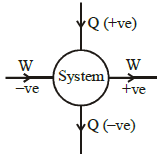•  For closed system and reversible process work done is calculated by ∫pdV.
•  In Cyclic Process:
•  For point function total change in property, in the cycle is zero.

ΔV=0, ΔP=0, ΔT=0, ΔU=0, Δh=0

• For path function net change in the cycle can may or may not be zero.

Work done in various reversible processes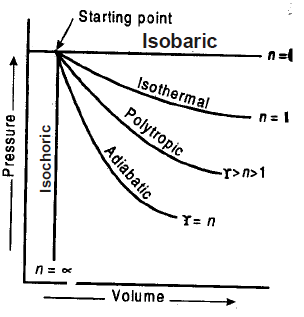Process Work done
(i) Constant pressure (isobaric) w1-2 = p(v2 – v1) = mR (T2 – T1)
(ii) Constant volume (isochoric) w1–2 = 0
(iii) Constant Temperature (isothermal)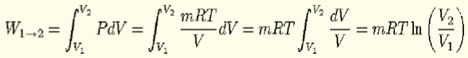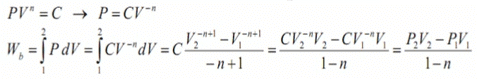n = polytropic const.
m = no. of moles

•  Mean effective pressure (Pm)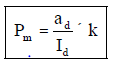Id — length of the p–v diagram along v–axis
ad — area of the p–v diagram
k — Spring constant

•  Indicated power (for two stroke engine)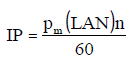• Indicated power (for four stroke engine)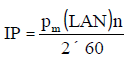Brake power (BP) =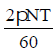Mechanical efficiency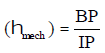L — stroke of the piston
A — cross sectional area of the cylinder(πD2/4)

N — r.p.m of the crank shaft
n — number of cylinders
T — Torque on the crank shaft

• Mass Flow rate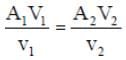V — Velocity of flow
v — Specific volume
A — Cross sectional area

Flow work : The flow work in an open system represents the energy transfered across the system boundary as a result of the energy imparted to the fluid by a pump, blower or compressor to make the fluid across the control volume. It is analogous to
displacement work. Flow work per unit mass Wflow = PV
P — pressure
v — specific volume

•  Expansion of a gas against vacuum is called free expansion.
•  There is no work transfer involved in free expansion.
•  Heat flow out of a system is taken as negative while heat flow into a system is taken as positive.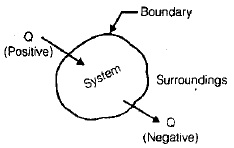• Heat like work is also a path function so is inexact or imperfect differential.

Specific heat c =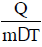Heat capacity C = m.c

• For solids, specific heat does not depend on the process.
• The latent heat of fusion is the amount of heat transferred to melt unit mass of solid into liquid or to freeze unit mass of liquid to solid at a constant pressure and temperature.

FQ = mlF
lF = Latent heat of fusion
• The latent heat of vaporisation is the amount of heat transferred to vaporize unit mass of liquid into vapour or condense unit mass of vapour into liquid at a constant pressure and temperature.

The document Work & Heat Transfer - Notes | Study Mechanical Engineering SSC JE (Technical) - Mechanical Engineering is a part of the Mechanical Engineering Course Mechanical Engineering SSC JE (Technical).
All you need of Mechanical Engineering at this link: Mechanical Engineering

## Mechanical Engineering SSC JE (Technical)

6 videos|97 docs|55 tests

## Mechanical Engineering SSC JE (Technical)

6 videos|97 docs|55 tests

Track your progress, build streaks, highlight & save important lessons and more!

,

,

,

,

,

,

,

,

,

,

,

,

,

,

,

,

,

,

,

,

,

;